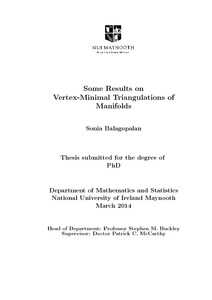Some Results on Vertex-Minimal Triangulations of Manifolds

Balagopalan, Sonia (2014) Some Results on Vertex-Minimal Triangulations of Manifolds. PhD thesis, National University of Ireland Maynooth.Previewmore...Add this article to your Mendeley library

Abstract

This thesis presents some results on vertex-minimal (simplicial) triangulations of manifolds. We are interested in triangulations that have nice geometric and combinatorial properties. In the first chapter, we list some defnitions used throughout the thesis. In the second chapter, we give an elementary construction of the Witt design on 22 points, and a combinatorial description of the only known vertex-minimal triangulation of real projective 4-dimensional space. We show that the 16-vertex complex we describe triangulates RP4 by constructing a 4-dimensional combinatorial sphere which can be easily seen to be a double cover of our complex. In the third chapter, we give two geometric constructions of the 16-vertex RP4. In the fourth chapter, we give a purely combinatorial description of a 15-vertex triangulation of an 8-manifold that has the same cohomology as the quaternionic projective plane HP2, and is conjectured to be homeomorphic to HP2.

Item Type: Thesis (PhD) Vertex-Minimal Triangulations; Manifolds; Faculty of Science and Engineering > Mathematics and Statistics 5024 IR eTheses 12 Jun 2014 14:45Item control page